JEE  >  True/False: Electrostatics | JEE Advanced

# True/False: Electrostatics - JEE Advanced - Physics 35 Year Past year Papers JEE Main & Advanced

True/False

Q.1. The work done in carrying a point charge from one point to another in an electrostatic field depends on the path along which the point charge is carried.     (1981- 2 Marks)

Ans. F

Solution. Electrostatic force is conservative in nature, therefore work done is path independent.

Q.2. Two identical metallic spheres of exactly equal masses are taken. One is given a positive  charge Q coulombs and the other an equal negative charge. Their  masses after charging are different.    (1983 - 2 Marks)

Ans. T

Solution. The metallic sphere which gets negatively charged gains electrons and hence its mass increases.

The metallic sphere which gets positively charged loses electrons and hence its mass decreases.

Q.3. A small metal ball is suspended in a uniform electric field with the help of an insulated thread. If high energy X-ray beam falls on the ball, the ball will be deflected in the direction of the field. (1983 - 2 Marks)

Ans. T

Solution. When high energy X-ray beam falls, it will knock out electrons from the small metal ball making it positively charged.

Therefore the ball will be deflected in the direction of electric field.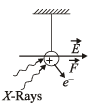Q.4. Two protons A and B are placed in between the two plates of a parallel plate capacitor charged to a potential difference V as shown in the figure. The forces on the two protons are identical.     (1986 - 3 Marks)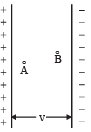Ans. T

Solution. The electric field produced between th e parallel plate capacitor is uniform. The force acting on charged particle placed in an electric field is given by F = qE.

In the case of two protons, q and E are equal and hence force will be equal.

Q.5. A ring of radius R carries a uniformly distributed charge + Q.

A point charge – q is placed on the axis of the ring at a distance 2R from the centre of the ring and released from rest. The particle executes a simple harmonic motion along the axis of the ring.     (1988 - 2 Marks)

Ans. F

Solution. KEY CONCEPT : Force on charge (– q) due to small charge dq situated at length dℓ is given by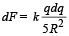Resolving this force into two parts dFcos θ and dFsin θ as shown in figure.

If we take another diametrically opposite length d, the charge on it being dq. Then the force on charge (– q) by this small charge dq will be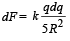Again resolving this force, we find dFsin q components of the two forces cancel out and dFcos q component adds up.

∴ The total force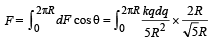Charge on length 2πR = Q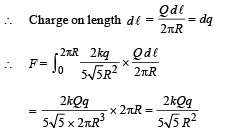This is not an equation of simple harmonic motion.

Q.6. An electric line of forces in the x – y plane is given by the equation x2 + y2 = 1. A particle with unit positive charge, initially at rest at the point x = 1, y = 0 in the x – y plane, will move along the circular line of force.     (1988 - 2 Marks)

Ans. F

Solution. For a particle to move in circular motion, we need a centripetal force which is not available.

The statement is false.

The document True/False: Electrostatics | JEE Advanced | Physics 35 Year Past year Papers JEE Main & Advanced is a part of the JEE Course Physics 35 Year Past year Papers JEE Main & Advanced.
All you need of JEE at this link: JEE

## FAQs on True/False: Electrostatics - JEE Advanced - Physics 35 Year Past year Papers JEE Main & Advanced

 1. What is electrostatics?Ans. Electrostatics is a branch of physics that deals with the study of stationary electric charges and the forces and fields associated with them.
 2. What are some real-life applications of electrostatics?Ans. Electrostatics has various applications in our daily lives. Some examples include the operation of photocopiers, laser printers, air purifiers, and electrostatic precipitators used in smokestacks to remove pollutants.
 3. How does electrostatic force differ from gravitational force?Ans. Electrostatic force is much stronger than gravitational force. It acts between charged particles, while gravitational force acts between any two objects with mass. Moreover, electrostatic force can be both attractive and repulsive, while gravitational force is always attractive.
 4. What is Coulomb's law?Ans. Coulomb's law states that the force between two point charges is directly proportional to the product of their magnitudes and inversely proportional to the square of the distance between them. It can be mathematically represented as F = k(q1q2/r^2), where F is the electrostatic force, q1 and q2 are the magnitudes of the charges, r is the distance between them, and k is the electrostatic constant.
 5. How does the electric field relate to electrostatics?Ans. The electric field is a concept used in electrostatics to describe the influence exerted by a charged object on other charged objects in its vicinity. It is a vector quantity that represents the force experienced by a unit positive charge placed in the field. The electric field is directly proportional to the charge creating it and inversely proportional to the square of the distance from the charge.

## Physics 35 Year Past year Papers JEE Main & Advanced

110 docs|49 tests

## Physics 35 Year Past year Papers JEE Main & Advanced

110 docs|49 tests
Signup to see your scores go up within 7 days! Learn & Practice with 1000+ FREE Notes, Videos & Tests.
10M+ students study on EduRev
Track your progress, build streaks, highlight & save important lessons and more!(Scan QR code)
Related Searches

,

,

,

,

,

,

,

,

,

,

,

,

,

,

,

,

,

,

,

,

,

;